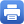Columbia Home
Geometry and Analysis

Geometry and analysis are particularly vibrant at Columbia University. These are vast fields, with myriad facets reflected differently in the leading mathematics departments worldwide. At Columbia, they are closely intertwined, with partial differential equations as the common unifying thread, and fundamental questions from several complex variables, algebraic geometry, topology, theoretical physics, probability, and applied mathematics as guiding goals.

The theory of partial differential equations at Columbia is practically indistinguishable from its analytic, geometric, or physical contexts: the d-bar-equation from several complex variables and complex geometry, real and complex Monge-Ampère equations from differential geometry and applied mathematics, Schrodinger and Landau-Ginzburg equations from mathematical physics, and especially the powerful theory of geometric evolution equations from topology, algebraic geometry, general relativity, and gauge theories of elementary particle physics. Of particular interest are manifestations of non-linearity and curvature, long-time behavior and inherently non-perturbative aspects, formation of singularities, generalized and viscosity solutions, and global obstructions to the existence and regularity of solutions. Although real and complex differential geometry can be quite different in orientation – the latter having closer ties with algebraic geometry and number theory – both are strongly represented at Columbia.

Other less analytic aspects of the theory of partial differential equations also thrive at Columbia. Of particular importance is the theory of solitons and integrable models, with their hidden symmetries and deep geometric structures, and stochastic differential equations, with the ever growing manifestations of random phenomena.

The senior faculty in geometry and analysis at Columbia at the present time consists of Panagiota Daskalopoulos (harmonic analysis and PDE), Richard Hamilton (differential geometry and PDE), Melissa Liu (symplectic geometry and general relativity), Duong H. Phong (complex analysis and mathematical physics), Mu-Tao Wang (differential geometry and PDE), and Ovidiu Savin (PDE). Closely affiliated are Andrei Okounkov (representation theory), and Ioannis Karatzas (probability and stochastic DE’s). From its PDE and differential geometry core, the group branches out for strong interactions with other groups in the department and the university, notably the groups in algebraic geometry, topology, number theory, string theory, and applied mathematics.Print this page